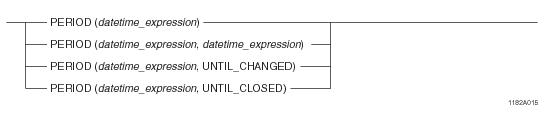# 15.00 - Period Value Constructor - Teradata Database

## Teradata Database SQL Functions, Operators, Expressions, and Predicates

Product
Release Number
15.00
Content Type
Programming Reference
Publication ID
B035-1145-015K
Language
English (United States)

## Period Value Constructor

### Purpose

Initializes an instance of a Period data type.

### Syntaxwhere:

 Syntax element... Specifies... datetime_expression any expression that evaluates to a DATE, TIME, or TIMESTAMP data type. UNTIL_CHANGED a DATE or TIMESTAMP value that is considered to be forever or until it is changed. For PERIOD(DATE) types, UNTIL_CHANGED has a value of DATE '9999-12-31'. For PERIOD(TIMESTAMP[(n)] [WITH TIME ZONE]) types, UNTIL_CHANGED has a value of TIMESTAMP '9999-12-31 23:59:59.999999 00:00'(with the precision truncated to the precision of the beginning bound and the time zone omitted if the beginning bound does not have a time zone). UNTIL_CHANGED supports derived periods. See “IS UNTIL_CHANGED/IS NOT UNTIL_CHANGED” on page 1071. UNTIL_CLOSED an ending bound for the Period value of a temporal table transaction-time column that indicates that the row is an open row. UNTIL_CLOSED has a data type of TIMESTAMP(6) WITH TIME ZONE and a value of TIMESTAMP '9999-12-31 23:59:59.999999+00:00'. For more information about temporal tables, see Temporal Table Support. UNTIL_CLOSED supports derived periods. See “IS UNTIL_CLOSED/IS NOT UNTIL_CLOSED” on page 1073.

### Result Value

The following rules apply to the result value:

• If the beginning or ending bound is NULL, or both the bounds are NULL, the result is NULL.
• If the beginning and ending bounds are NULL or if the beginning bound is NULL and the ending bound is UNTIL_CHANGED, then the type of the period defaults to PERIOD(TIMESTAMP(0)).
• If only the beginning bound is specified, the result ending bound is the beginning bound plus one granule of the result element type. If the result ending bound exceeds or becomes equal to the maximum allowed DATE or TIMESTAMP value for result data type of PERIOD(DATE) or PERIOD(TIMESTAMP(n) [WITH TIME ZONE]), respectively, an error is reported.
• If an ending bound is specified as a value expression and the beginning bound and ending bound have different precisions, the result precision is the higher of the two precisions. Otherwise, the result precision is the precision of the beginning bound.
• UNTIL_CHANGED sets the result ending element to a maximum DATE or TIMESTAMP value depending on the data type of the beginning bound. If the data type of the beginning bound is TIMESTAMP(n) WITH TIME ZONE, the result ending element is set to the maximum TIMESTAMP(n) WITH TIME ZONE value at UTC (that is, the time zone displacement for the ending bound is INTERVAL '00:00' HOUR TO MINUTE).
• If the beginning bound or the ending bound or the beginning and ending bounds include a time zone value, and the ending bound is not UNTIL_CHANGED, the result data type is WITH TIME ZONE. If only one of the bounds includes a time zone value, the time zone field of the other is set to the current session time zone displacement. If both bounds include time zone values, the result bounds include the corresponding time zone value.
• The result Period data type has an element type that is the same as the DateTime data type of the beginning bound except with the precision and time zone as defined previously.
• The handling of leap seconds for Period data types with TIME and TIMESTAMP element types is as follows. If the value for the beginning or ending bound contains leap seconds, the seconds portion gets adjusted to 59.999999 with the precision truncated to the result precision. During this process, if the beginning and ending bounds are the same, an error is reported.
• ### Usage Rules

The following rules apply to the Period value constructor:

• The beginning bound must have a DateTime data type and, if an ending bound is specified, the data types of the beginning and ending bounds must be comparable.
• The ending bound where the data type of the beginning bound is DATE or TIMESTAMP can be set to UNTIL_CHANGED.
• If the ending bound is set to UNTIL_CLOSED, the following must be true:
• The data type of the beginning bound value must be comparable with TIMESTAMP(6) WITH TIME ZONE.
• The constructor is only valid in an assignment operation where the target column to which the result is assigned is a transaction-time column.
• Because the only way to set the value of a transaction-time column is by using nontemporal DML, the constructor is only valid in a nontemporal DML statement.
• Teradata Database reports an error if any of the following are true:
• UNTIL_CHANGED is specified for the beginning bound.
• The result beginning bound is greater than or equal to the result ending bound.
• The data types of the beginning and ending bounds are not comparable.
• UNTIL_CHANGED is specified for the ending bound and the data type of the beginning bound is TIME(n) [WITH TIME ZONE].
• UNTIL_CLOSED is specified for the beginning bound.
• ### Example

In the following example, assume t1 is a table with an INTEGER column c1 and a PERIOD(DATE) column c2 and t2 is a table with an INTEGER column a and two DATE columns b and c.

This example shows the Period value constructor used in two INSERT statements.

`INSERT INTO t1`
`  VALUES (1, PERIOD(DATE '2005-02-03', DATE '2006-02-04'));`
`INSERT INTO t1 SELECT a, PERIOD(b, c) FROM t2;`